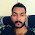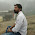# C++ Program To Print A Pascal's Triangle

Problem :- Write A C++ Program To Print A Pascal Triangle .

Logic :- In mathematics, Pascal's triangle is a triangular array of the binomial coefficients. In the Western world, it is named after French mathematician Blaise Pascal, although other mathematicians studied it centuries before him in India, Persia (Iran), China, Germany, and Italy. Wikipedia

For printing Pascal Triangle we need to understood how to print pascal triangle the given gif shows how to print pascal triangle

Now we know the how pascal triangle works and i also want to tell you a fantastic Fact about Pascal Triangle .

Pascal Triangle helps in Probability to find outcome
Sum of the row in Pascal triangle is Power of 2
Pascal triangle Also help to Print Fibonacci Series
If you really want to print Pascal Number just do one thing
11^0=1                              (1)
11^1=1 1                          (11)
11^2=1 2 1                      (121)
11^3=1 3 3 1                  (1331)
11^4=1 4 6 4 1              (14641)
11^5=1 5 1 0 1 0 5 1    (15101051)
All Are Pascal Number

Try Yourself C Program For Print A Left Pascal Triangle Using Number

Solution :-

#include<iostream>
using namespace std;
long int f(int);
int main()
{
long int j,n,x;

while(1)
{
cout<<"\n\nEnter The Number of line : ";
cin>>n;

for(int i=0;i<n;i++)
{
cout<<"\n";
x=0;
for(j=1;j<=2*n;j++)
if(j>=n-i&&j<=n)
{
if((j-n+i+1)%2==0)
cout<<"  ";
else
{
cout<<f(i)/(f(i-x)*f(x));
x++;
if(j==n)
x--;
}
}
else if(j<=n+i&&j>n)
{
if((n+i-j+1)%2==0)
cout<<"  ";
else
{
--x;
cout<<f(i)/(f(i-x)*f(x));
}
}
else
cout<<"  ";
}
}
return 0;
}

long int f(int x)
{
long int i,a=1;

for(i=1;i<=x;i++)
a=a*i;
return a;
}

Output:-

1.1.